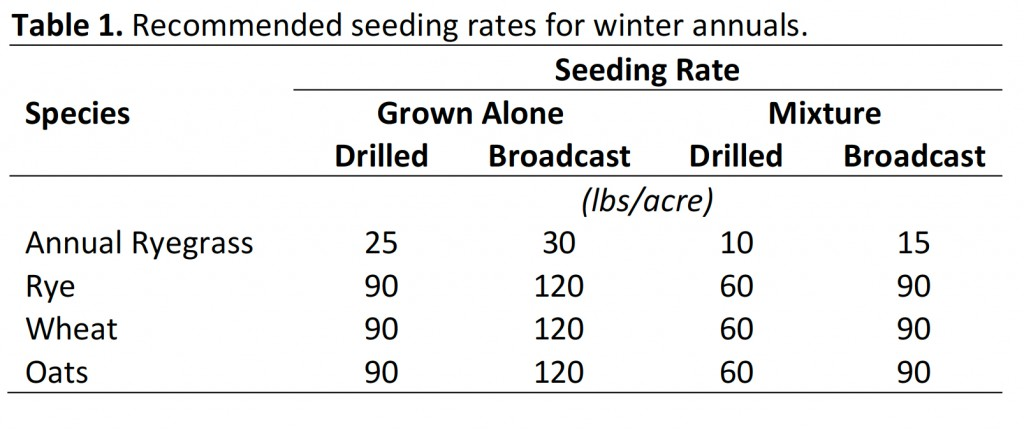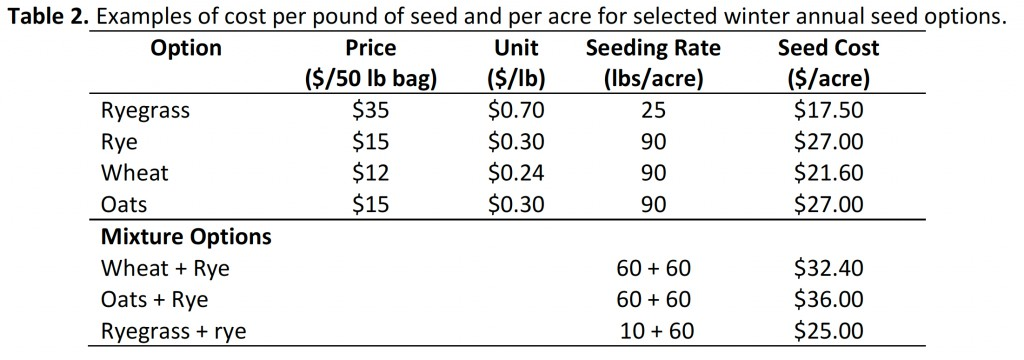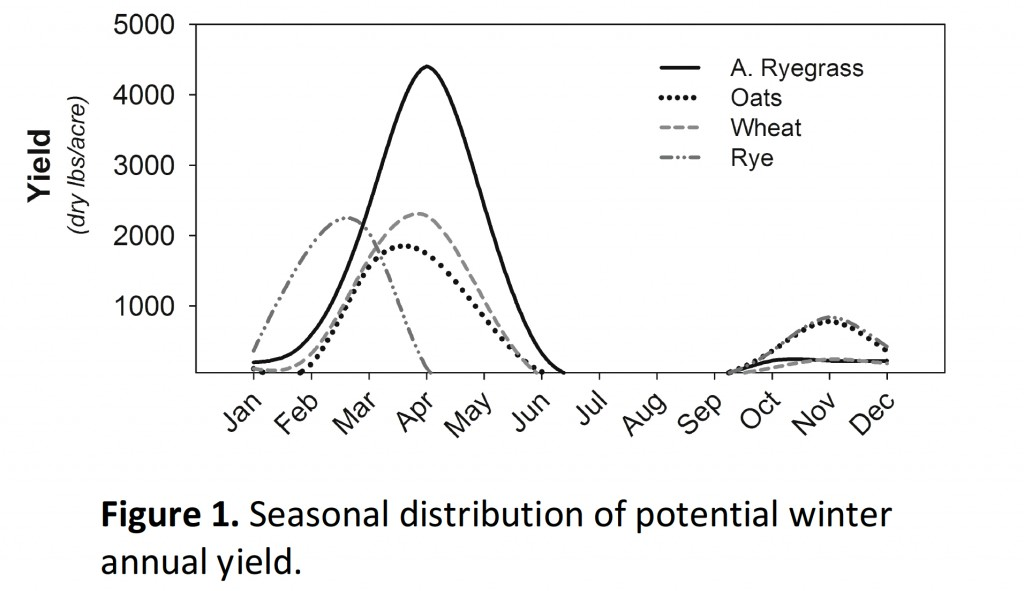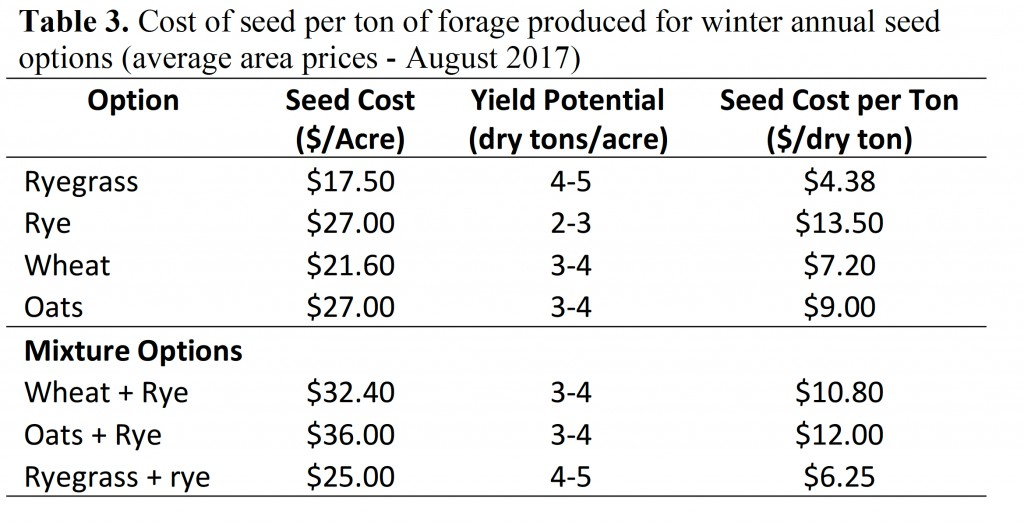# Winter Annual Seed Costs: What is the Best Deal?

by Adam Speir and Dennis HancockHumans are creatures of habit, and farmers are no different.  We tend to make purchase decisions by looking at the immediate ticket price without considering long term costs. When considering winter annual seed costs, consider not only upfront seed costs, but also the overall costs and benefits of those seed choices. We’ll discuss a few examples that will show you how to determine 1) Cost per pound of seed, 2) Cost of seed per acre planted, and 3) Cost of seed per pound of forage produced.  These exercises will hopefully help you better determine what will provide you the best return on investment.Before doing so, we need to make a few assumptions regarding seeding options and rates.  Annual ryegrass, cereal rye, oats, and wheat are some of the most common winter annual choices for forage producers in Georgia.  Seeding rates vary for these depending on seeding method and if they are planted individually or in a mixture (Table 1).  We will assume lower seeding rates in the examples used in this exercise.

Part 1 – Determining Cost of Seed per Pound.

Cost of seed will likely vary depending on location and availability.  Table 2 shows the price per pound for various options currently (August 2017).  This price per pound is easily calculated by dividing the price of the seed by the number of pounds in a bag.  For example, if a 50-lb bag of annual ryegrass costs \$35, then \$35/50 lbs will equal a cost of \$0.70 per pound.  In the table, one can see that the cost (per pound) of annual ryegrass would be highest while wheat is the lowest. But, that is not the full story, as it does not consider differences in seeding rate.

Part 2 – Determining Cost of Seed per Acre

As indicated in Table 1, the seeding rate for small grains such as wheat or oats is much higher than that for annual ryegrass.  The seeding rate per acre plays a large part in getting a better idea of one’s unit cost.  Table 2 shows that while ryegrass is the most expensive seed per pound, it is the least expensive option on a per acre basis.  There are also three different seed mix examples included to help you see where these options rank.  To calculate these, you simply take the seeding rate and multiply by price per pound.  For example, if cereal rye has a seeding rate of 90 pounds per acre and a cost of \$0.30 per pound, then 90 lbs./acre x \$0.30 = \$27 per acre.  For seed mixture options, you simply perform the same calculation and add the two together (i.e. wheat + rye @60 lbs./acre each: (60 x \$0.24) + (60 x \$0.30) = \$14.40 + \$18 = \$32.40. While there are three different mixes specifically mentioned in this example, working with your county Extension agent can help you determine if other mixes are appropriate in your situation.Part 3 – Determining Cost of Seed per Pound of Forage Produced

Finally, and most importantly, it is crucial to understand how the yield potential of these different forage options impact the final unit cost.  As you may know, not all forages are created equal.  And while each of these different choices may have their place in a farm operation, understanding the potential of their yield production helps us make the best management decisions possible.  Figure 1 shows the year-long yield potential of each of these seed options.  Table 3 shows the possible yield potential of these forages and cost per pound of forage produced.  When mixing different species, while the distribution of the two species may provide longer grazing availability, the total yield potential is typically limited to the highest yielding crop in the mix.  In this example, I am assuming the lower range of yield expectations.After going through these basic calculations, we can see that while the initial sticker shock of the cost of ryegrass seed is high, it is still the best deal in terms of seed cost per ton of forage. There are, of course, other costs to consider, including differences in fertilizer, establishment, and other variable costs (for the latest winter annual budgets, visit: http://www.caes.uga.edu/departments/ag-econ/extension/budgets.html). Nonetheless, this exercise illustrates the point that one should compare input costs in the context of the output they will get in return.

Adam Speir, Madison Co. County Extension Director and UGA Forage Team Member

Dr. Dennis Hancock, Assoc. Prof. and State Forage Ext. Agronomist, Crop and Soil Sciences Dept., UGA